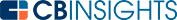# What is Intrinsic Value?

Intrinsic value is a measurement of an asset’s actual worth, regardless of its market value. In order to understand this concept, it is vital to understand the difference between intrinsic value and market value.

A stock’s market value is the price it would sell on the open market. This metric is influenced by the investment community and changes based on factors like supply and demand, operating conditions, and the broader economic landscape. In contrast, market value does not reflect the actual value of a company.

Intrinsic value is an estimate of a company’s worth without the investment community’s influence. Analysts use factors like business plans, market analyses, and financial statements to determine the intrinsic value of a company. Intrinsic value is calculated to reflect the actual value of a company

## How is a stock’s intrinsic value calculated?

Because intrinsic value estimates future cash flows and risk, there’s no universally accepted standard for calculating it. A stock’s intrinsic value is typically calculated through valuation models created by financial analysts.

These models use quantitative, qualitative, and perceptual factors to help determine a company’s intrinsic value. Discounted cash flow, dividend discount, and residual income models are three common ways to calculate a stock’s intrinsic value.

A discounted cash flow (DCF) analysis uses a company’s expected future cash flows to determine its intrinsic value. The DCF formula is:The dividend discount model discounts future dividend payments back to their current value to calculate intrinsic value. Its formula is:Where:

EDPS = expected dividend per share

CCE = cost of capital equity

DGR = dividend growth rate

The residual income model combines a company’s discounted residual income with its present book value per share to estimate intrinsic value. This model’s formula is:## Why is intrinsic value important?

Investors who accurately estimate a company’s intrinsic value can determine if it’s under- or overvalued by the market.

A stock trading under its intrinsic value presents an opportunity for investors to purchase it at a discount. An investor with this knowledge can conclude the stock’s price should be higher than the market gives it credit for. A stock trading at a higher price than its intrinsic value is inflated. This is less attractive to investors because it means the stock is valued higher than its worth.

Understanding intrinsic value is thus crucial when deciding whether a stock is a good investment with potential for high returns.

## What else does intrinsic value apply to?

In addition to pricing stocks, intrinsic value helps with options pricing. The intrinsic value of an option is the difference between the strike price and the price of the underlying stock. This shows an investor how much in the money the option is.

In the money options have a positive payoff to investors. If an option is out of the money, its intrinsic value equals \$0. The intrinsic value formula for options varies depending on if the option is a put or a call.

For an in the money (ITM) call option:

Intrinsic value = current stock price - strike price

For an in the money (ITM) put option: intrinsic value = strike price – current stock price.

Intrinsic value = strike price - current stock price

For example, imagine that a stock is trading at \$25 and a call option on this stock’s strike price is \$15. This option’s intrinsic value therefore equals \$10.

If instead, this had been a put option with a strike price equaling \$40, its intrinsic value would be \$15. A call option greater than \$25, or a put option below \$25 would be out of the money, causing intrinsic value to equal \$0.

The intrinsic value of an option is important because it shows investors the profit amount existing within a contract. However, it leaves out factors like time value and premium payments. These must be factored in to fully understand the total profit an investor will make.

Intrinsic value is the estimated value of a stock’s worth. It does not factor in the value of the stock on the open market. While there isn’t one specific way to calculate it, common calculation models include discounted cash flow, dividend discount, and residual income.

Investors need to estimate intrinsic value to help them determine if a stock is worth investing in. Intrinsic value can also be used to find how much in the money an option is.

You may also like# www.cbinsights.com